## Friday 3 July 2020

### Do non binary forecasts work?

This is a post about forecasts in trading systems. A forecast is a calibrated expectation for future risk adjusted returns. In more layman like terms, it is a measure of how confident we are about a bullish (positive forecast) or bearish (negative forecast).

Perhaps it is easiest to think about forecasts if we compare them to what is not: a forecast is non binary. A binary trading system will decide whether to go long, or short, but it does not get more granular than that. It will buy, or sell, some fixed size of position. The size of the position may vary according to various factors such as risk or account size (enumerated in this recent post) but importantly it won't depend on the level of forecast conviction.

In my two books on trading ('Systematic' and 'Leveraged' Trading) I confidently stated that non binary forecasts work: in other words that you should scale your positions according to the conviction of your forecasts, and doing so will improve your risk adjusted returns compared to using binary forecasts.

I did present some evidence for this in 'Leveraged Trading', but in this post I will go into a lot more detail of this finding, and explore some nuances.

This will be the first in a series of four broadly related posts. The second post will explore volatility forecasting, and whether it improving it can improve forecasting.

In the third post I explore the issue of whether it makes sense to fix your expected portfolio risk (a question that was prompted by a comment on a recent post I did on exogenous risk management).  This is related to forecasting, because the use of forecasts imply that you should let your expected risk vary according to how strong your forecasts are. If forecasting works, then fixing your risk should make no sense.

The final post (as yet unwritten) will be about the efficient use of capital for small traders. If forecasts work, then we can use capital more efficiently by only taking positions in instruments with large forecasts. I explored this to some degree in a previous post where I used a (rather hacky) non linear scaling to exploit this property. I have recently had an idea for doing this in a fancier way that will allow very large portfolios with very limited capital. This might end up being more than one post... and may take a while to come out.

Let us begin.

<UPDATE 6th July: Added 'all instruments' plots without capping>

## Forecasts and risk adjusted returns

Econometrics 101 says that if you want to see wether there is a relationship between two variables you should start off by doing some kind of scatter plot. Forecasts try and predict future risk adjusted returns, so we'll plot the return for the N days, divided by the daily volatility estimate for the return. We get N days by first estimating the average holding period of the forecast. On the x-axis we'll plot the forecast, scaled to an average absolute value of 10.

from syscore.pdutils import turnover
`import numpy as np`
`from systems.provided.futures_chapter15.basesystem import futures_system`
`system = futures_system()`
`def get_forecast_and_normalised_return(instrument, rule):    forecast = system.forecastScaleCap.get_scaled_forecast(instrument, rule)    # holding period    Ndays = int(np.ceil(get_avg_holding_period_for_rule(forecast)))    raw_price = system.data.get_raw_price(instrument)    ## this is a daily vol, adjust for time period    returns_vol = system.rawdata.daily_returns_volatility(instrument)    scaled_returns_vol = returns_vol * (Ndays**.5)    raw_daily_price = raw_price.resample("1B").last().ffill()    ## price Ndays in the future    future_raw_price = raw_daily_price.shift(-Ndays)    price_change = future_raw_price - raw_daily_price     # these normalised change will have E(standard deviation) 1    normalised_price_change = price_change / scaled_returns_vol.ffill()    pd_result = pd.concat([forecast, normalised_price_change], axis=1)    pd_result.columns = ['forecast', 'normalised_return']    pd_result = pd_result[:-Ndays]    return pd_resultdef get_avg_holding_period_for_rule(forecast):    avg_annual_turnover = turnover(forecast, 10)    holding_period = 256 / avg_annual_turnover    return holding_periodLet's use the trading rule from chapter six of "Leveraged Trading", EWMAC 16,64*; and pick an instrument I don't know Eurodollar**. * That's a moving average crossover between two exponentially weighted moving averages, with a 16 day and a 64 day span respectively** Yes I've cherry picked this to make the initial results look nice and bring out some interesting points, but I will be doing this properly across my entire universe of futures later`
`instrument="EDOLLAR"rule = "ewmac16_64"pd_result = get_forecast_and_normalised_return(instrument, rule)pd_result.plot.scatter('forecast', 'normalised_return')`X-Axis forecast, Y-axis subsequent risk adjusted return over average holding period of 17 weekdays
That is quite pretty, but not especially informative. It's hard to tell whether the trading rule even works, i.e. is a positive forecast followed by a positive return over the next 17 business days (which happens to be the holding period for this rule), and vice versa? We can check that easily enough by seeing what the returns are like conditioned on the sign of the forecast:
`pos_returns = pd_result[pd_result.forecast>0].normalised_returnneg_returns = pd_result[pd_result.forecast<0].normalised_returnprint(pos_returns.mean())print(neg_returns.mean())print(stats.ttest_ind(pos_returns, neg_returns, axis=0, equal_var=True))`

The returns, conditional on a positive forecast, are 0.21 versus 0.02 for a negative forecast. The t-test produces a T-statistic of 7.6, and the p-value is one of those numbers with e-14 at the end of it so basically zero. Incidentally there were more positive forecasts than negative by a ratio of ~2:1, as Eurodollar has generally gone up.

## Is the response of normalised return linear or binary?

So far we have proven that the trading rule works, and that a binary trading rule would do just fine thanks very much. But I haven't yet checked whether taking a larger forecast would make more sense. I could do a regression, but that could produce the same result if the relationship was linear or if it was binary (and the point cloud above indicates that the R^2 is going to be pretty dire in any case).

Let's do the above analysis but in a slightly more complicated way:

`from matplotlib import pyplot as pltdef plot_results_for_bin_size(size, pd_result):    bins = get_bins_for_size(size, pd_result)    results = calculate_results_for_bins(bins, pd_result)    avg_results = [x.mean() for x in results]    centre_bins = [np.mean([bins[idx], bins[idx - 1]]) for idx in range(len(bins))[1:]]    plt.plot(centre_bins, avg_results)`
`    ans = print_t_stats(results)    return ans`
`def print_t_stats(results):    t_results = []    for idx in range(len(results))[1:]:        t_stat = stats.ttest_ind(results[idx], results[idx-1], axis=0, equal_var=True)        t_results.append(t_stat)        print(t_stat)`
`    return t_resultsdef get_bins_for_size(size, pd_result):    positive_quantiles = quantile_in_range(size, pd_result, min=-0.001)    negative_quantiles = quantile_in_range(size, pd_result, max=0.001)    return negative_quantiles[:-1]+[0.0]+positive_quantiles[1:]def quantile_in_range(size, pd_result, min=-9999, max=9999):    forecast = pd_result.forecast    signed_distribution = forecast[(forecast>min) & (forecast<max)]    quantile_ranges = get_quantile_ranges(size)    quantile_points = [signed_distribution.quantile(q) for q in quantile_ranges]    return quantile_pointsdef get_quantile_ranges(size):    quantile_ranges = np.arange(0,1.0000001,1.0/size)    return quantile_rangesdef calculate_results_for_bins(bins, pd_result):    results = []    for idx in range(len(bins))[1:]:        selected_results = pd_result[(pd_result.forecast>bins[idx-1]) &  (pd_result.forecast < bins[idx])]        results.append(selected_results.normalised_return)    return results`
Typing plot_results_for_bin_size(1, pd_result) will give the same results as before, plotted on the worlds dullest graph: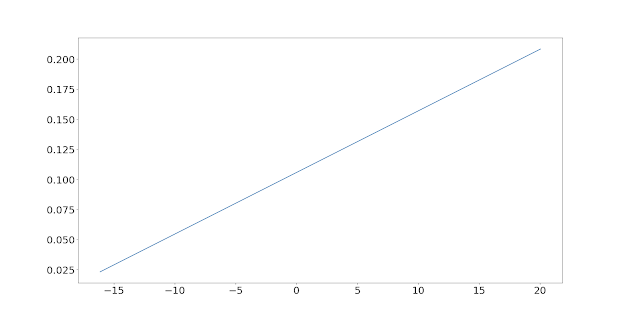Forecast and subsequent risk adjusted return for ewmac16_64 trading rule for Eurodollar. Mean risk adjusted return for two buckets, conditioned on sign of forecast.

Ttest_indResult(statistic=7.614065523409865, pvalue=2.907839550447572e-14)

Now let's up the ante, and use a bin size of 2, which means plotting 4 'buckets'. This means we're looking at normalised returns, conditional on forecast values being in the following ranges: [-32.3,-6.6], [-6.6, 0], [0, 9.0], [9.0, 40.1]. These might seem random but as the code shows the positive and negative region have been split, and then split further into 2 'bins' with 50% of the data put in one sub-region and 50% in the next. Roughly speaking then 25% of the forecast values will fall in each bucket (although we know that is not the case because there are more positive than negative forecasts).

Each point on the plot shows the average return within a 'bucket' on the y-axis, with the x-axis point in the centre of the 'bucket'.Forecast and subsequent risk adjusted return for ewmac16_64 trading rule for Eurodollar. Mean risk adjusted return for 4 buckets, conditioned on sign and distributional points of forecast.

What crazy non-linear stuff is this? Negative forecasts sure are bad (although this is Eurodollar, and it normally goes up so not that bad), and statistically worse than any positive forecast. But a modestly positive forecast is about as good as a large positive forecast. We can see a little more detail with 12 buckets (bins=6):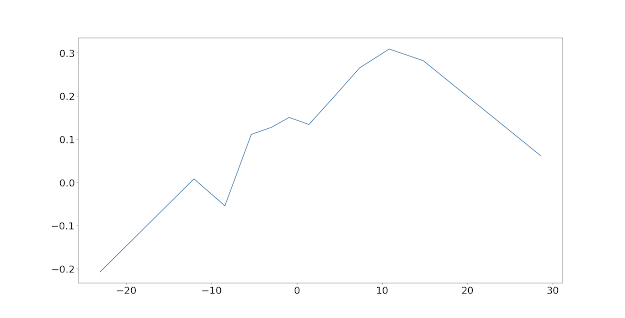Forecast and subsequent risk adjusted return for ewmac16_64 trading rule for Eurodollar. Mean risk adjusted return for 12 buckets, conditioned on sign and distributional points of forecast.

It's clear that, ignoring the wiggling around which is just noise, that there is indeed a roughly linear and fairly monotonic positive relationship between forecast and subsequent risk adjusted return, until the final bin (which represents forecast values of over 17). The forecast line reverts at the extremes.

This is a pretty well known effect in trend following, and there are a few different explanations. One is that trends tend to get exhausted after a while, so a very strong trend is due a reversal. Another is that high forecasts are usually caused by very low volatility (since forecasts are in risk adjusted space, low vol = high forecast), and very low vol has a tendency to mean revert at the same time as markets sharply change direction. Neithier of these explain why the result is assymetric; but in fact it's just that positive trends are more common in Eurodollar.

Here's the plot for Gold for example:Forecast and subsequent risk adjusted return for ewmac16_64 trading rule for Gold. Mean risk adjusted return for 12 buckets, conditioned on sign and distributional points of forecast.

There is clear reversion in both wings. And here's Wheat:Forecast and subsequent risk adjusted return for ewmac16_64 trading rule for Wheat. Mean risk adjusted return for 12 buckets, conditioned on sign and distributional points of forecast.

Here there is reversion for negative forecasts, but not for extreme positive forecasts.

## Introducing forecast capping

There are different ways to deal with this problem. At one extreme we could fit some kind of cubic spline to the points in these graphs, and create a non linear response function for the forecast. That smacks of overfitting to me.

There are slightly less mad approaches, such as creating a fixed sine wave type function or a linear approximation thereof. This has very few parameters but still leads to weird behaviour: when a trend reverses you initially increase your position unless you introduce hysteresis into your trading system (i.e. you behave differently when your forecast has been decreasing than when it is increasing).

A much simpler approach is to do what I actually do: cap the forecasts at a value of -20,20 (which is exactly double my target absolute value of 10). This also makes sense from a risk control point of view.

There are some other reasons for doing this, discussed in both my books on trading.

We just need to change one line in the code:
`forecast = system.forecastScaleCap.get_capped_forecast(instrument, rule)`

And here is the revised plot for Eurodollar with a bin size of 2:Capped forecast and subsequent risk adjusted return for ewmac16_64 trading rule for Eurodollar. Mean risk adjusted return for 4 buckets, conditioned on sign and distributional points of forecast.

That's basically linear, ish. With bin size of 6: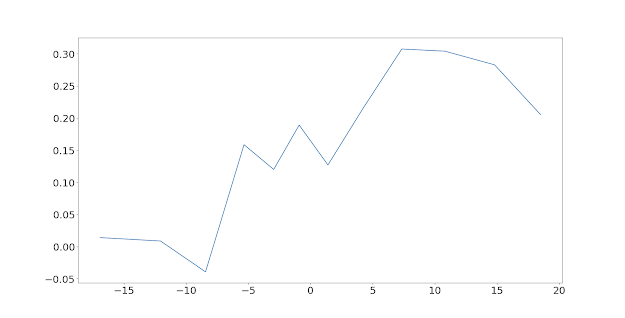Capped forecast and subsequent risk adjusted return for ewmac16_64 trading rule for Eurodollar. Mean risk adjusted return for 12 buckets, conditioned on sign and distributional points of forecast.

There is still a little reversion in the wings, but it's more symmetric and ignoring the wiggling there is clearly a linear relationship here. I will leave the problem of whether you should behave differently in the extremes for another day.

## Formally testing for non-binaryness

We'll focus on a bin size of 2 (i.e. a total of 4 buckets), which is adequate to see whether non binary forecasts make sense or not without having to look at a ton of numbers, many of which won't be significant (as the bucket size gets more granular, there is less data in each bucket, and so less significance).

We have the following possibilities drawn on the whiteboard. There are four points in each figure and thus 3 lines connecting them. From top to bottom:
• binary forecasts make sense
• linear forecasts make sense
• reverting forecasts make sense

In black are the results we'd get if the forecast worked (a positive relationship between normalised return and forecast). In red are the results if the forecast didn't work.

So we want a significantly positive slope for the first and third lines as in the middle black plot. But we'd also get that if we had a reverting incorrect forecast (bottom plot in red). So, I add an additional condition that a line drawn between the first and final points should also be positive.  We don't test the slope of the second line. This means that we'd ignore a response with an overall positive slope, but which has a slight negative 'flat spot' in the middle line.

Note: the first and third T-test comparisions are (by construction) between buckets of exactly the same size, which is nice.

The lines will be positive if the T-test statisics are positive (since they're one sided tests), and they will be significantly positive if the T-statistics give p-values of less than 0.05.

Let's modify the code so it reports the difference between the first and final points as well:

`def print_t_stats(results):    t_results = []    print("For each bin:")    for idx in range(len(results))[1:]:        t_stat = stats.ttest_ind(results[idx], results[idx-1], axis=0, equal_var=True)        t_results.append(t_stat)        print("%d %s " % (idx, str(t_stat))    print("Comparing final and first bins:")    t_stat = stats.ttest_ind(results[-1], results, axis=0, equal_var=True)    t_results.append(t_stat)    print(t_stat)    return t_results`

Here is the output for Eurodollar

>> plot_results_for_bin_size(2, pd_result)
For each bin:
Ttest_indResult(statistic=4.225710114631642, pvalue=2.44857998636189e-05)
Ttest_indResult(statistic=1.814973164207728, pvalue=0.06959073262053131)
Ttest_indResult(statistic=1.9782202453688769, pvalue=0.04795295675153716)
Comparing final and first bins:
Ttest_indResult(statistic=7.36610843915252, pvalue=2.1225843317794611e-13)

The key numbers are in bold: we can see that with a p-value of 0.0479 the third line just passes the test. But the first line and the overall slope tests are passed easily.

## Pooling data across instruments

Looking at one trading rule for one instrument is sort of pointless. We have quite a lot of price history for Eurodollar and we only just get statistical significance, for plenty of other instruments we wouldn't.

Earlier I openly admitted that I cherry picked Eurodollar; readers of Leveraged Trading will know that there are 8 futures markets in my dataset for which the test would definitely fail as this particular trading rule doesn't work (so we will be on one of the 'red line' plots).

I should probably have cherry picked a market with a clearer linear relationship, but I wanted to show you the funky reversion effect.

Checking each market is also going to result in an awful lot of plots! Instead I'm going to pool the results across instruments. Because the returns and forecasts are all risk adjusted to be in the same scale we can do this by simply stacking up dataframes. Note this will give a higher weight to instruments with more data.

`instrument_list = system.data.get_instrument_list()all_results = []for instrument_code in instrument_list:    pd_result = get_forecast_and_normalised_return(instrument_code, rule)    all_results.append(pd_result)all_results = pd.concat(all_results, axis=0)plot_results_for_bin_size(6, all_results)`
`Capped forecast and subsequent risk adjusted return for ewmac16_64 trading rule pooled across all instruments. Mean risk adjusted return for 12 buckets, conditioned on sign and distributional points of forecast.`
We didn't need to do this plot for the formal analysis, but I thought it would be instructive to show you that once the noise for individual instruments is taken away we basically have a linear relationship, with some flattening in the extremes for forecasts out of the range [-12,+12].

For the formal test we want to focus on the bin=2 case, with 4 points:
`plot_results_for_bin_size(2, all_results)`Capped forecast and subsequent risk adjusted return for ewmac16_64 trading rule pooled across all instruments. Mean risk adjusted return for 4 buckets, conditioned on sign and distributional points of forecast.

1 Ttest_indResult(statistic=10.359086377726523, pvalue=3.909e-25)
2 Ttest_indResult(statistic=13.502334211993352, pvalue=1.617e-41)
3 Ttest_indResult(statistic=15.974961084038702, pvalue=2.156e-57)
Comparing final and first bins:
Ttest_indResult(statistic=35.73832257341082, pvalue=3.421-e278)

Remember: we want a significantly positive slope for the first and third lines: yes without question. We also want a significantly positive slope between the first and final bins, again no problems here.

For the overall slope, I didn't even know python could represent a p-value that small in floating point. Apparently we can get down to 1.79e-308!

Note that if the first and third T-tests statistics were zero, that would indicate a binary rule would make sense. If they were negative, it would indicate reversion. Finally, if the final comparision between the last and first bins was negative, then the trading rule wouldn't work

I think we can all agree that for this specific trading rule, a non binary forecast makes sense.

## Testing all momentum rules

We can extend this to the other momentum rules in our armoury. For all of these I'm going to plot the bins =6 case with and without capping (because they're usually more fun to look at, and because <spolier alert> they show an interesting pattern in the tails which is more obvious without capping), and then analyse the bins=2 results with capping using the methodology above. Let's start at the faster end with ewmac2_8.

 Forecast without capping and subsequent risk adjusted return for ewmac2_8 trading rule, pooled across all instruments.Forecast with capping and subsequent risk adjusted return for ewmac2_8 trading rule, pooled across all instruments.

Notice that for this very fast trading rule (too expensive indeed to trade even for many futures), the behaviour in the tails is quite different: the slope definitely does not revert. We can see how people might be tempted to start fitting these response functions, but let's move on to the figures. We want all the T-statistics in bold to be positive and well above 2:

Ttest_indResult(statistic=3.62040542155758, pvalue=0.00029426)
Ttest_indResult(statistic=7.166027593239416, pvalue=7.761585-13)
Ttest_indResult(statistic=2.735993316153726, pvalue=0.006220)
Comparing final and first bins:
Ttest_indResult(statistic=12.883660014469108, pvalue=5.9049-38)

A resounding pass again. Here's ewmac4_8:Forecast without capping and subsequent risk adjusted return for ewmac4_16 trading rule, pooled across all instruments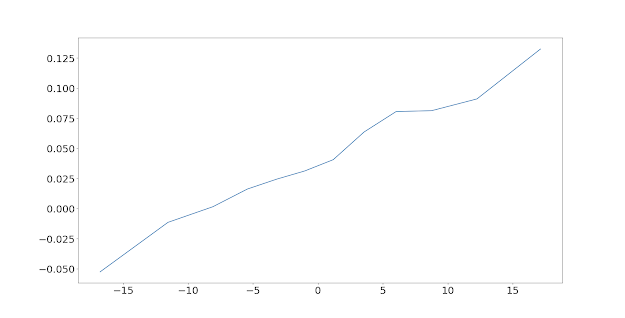Forecast with capping and subsequent risk adjusted return for ewmac4_16 trading rule, pooled across all instruments

We have a pretty smooth linear picture again. I won't bore you with the T-tests, which are all above 6.0 and positive.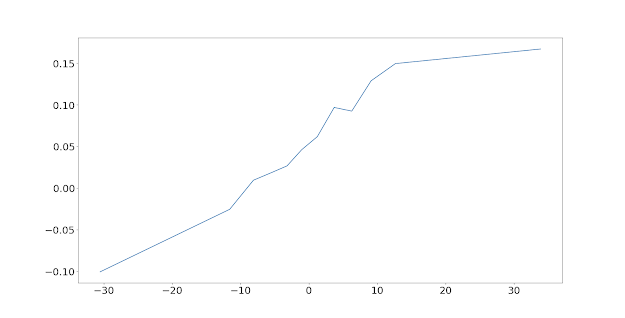Forecast without capping and subsequent risk adjusted return for ewmac8_32 trading rule, pooled across all instruments

 Forecast with capping and subsequent risk adjusted return for ewmac8_32 trading rule, pooled across all instruments

The t-statistics are now above 9. To keep things in order, and so you can see the pattern, here is the plot for ewmac16_64 (without capping, and with capping which we've already seen):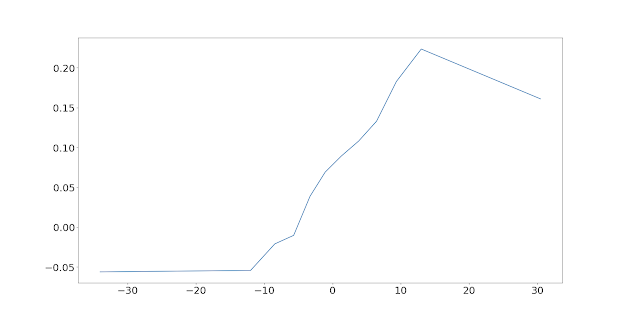Forecast without capping and subsequent risk adjusted return for ewmac16_64 trading rule, pooled across all instrumentsForecast with capping and subsequent risk adjusted return for ewmac16_64 trading rule, pooled across all instruments

Can you see the pattern? Look at the tails. In the very fastest crossover we saw a linear relationship all the way out. Then for the next two plots as the rule slowed down it became more linear. Now we're seeing the tails start to flatten, with strong reversion at the extreme bullish end (although this goes away with capping).

We already know this rule passes easily, so let's move on.Forecast without capping and subsequent risk adjusted return for ewmac32_128 trading rule, pooled across all instrumentsForecast with capping and subsequent risk adjusted return for ewmac32_128 trading rule, pooled across all instruments

Now there is a clear flat spot in both tails, so the pattern continues. Oh and the t-statistics are all well above 12.

One more to go:Forecast without capping and subsequent risk adjusted return for ewmac64_256 trading rule, pooled across all instrumentsForecast with capping and subsequent risk adjusted return for ewmac64_256 trading rule, pooled across all instruments

It's a pass in case you haven't noticed. And there is some evidence that the flattening/reversion is continuing to become more pronounced on the negative end.

Anyway to summarise, all EWMAC rules have non binary responses.

Now let's turn to the carry trading rule. Again I will plot the bin=6 case, and then analyse the statistics based on bin=2.Forecast without capping and subsequent risk adjusted return for carry trading rule, pooled across all instruments. Note that the x-axis has been truncated as carry signals without capping are in the range [-220,+160]Forecast with capping and subsequent risk adjusted return for carry trading rule, pooled across all instruments

That is pretty funky to say the least, and exploring it could easily occupy another post, but let's be consistent and stick to the methodology of analysing the bins=2 results:

Ttest_indResult(statistic=5.3244949302972255, pvalue=1.0147-07)
Ttest_indResult(statistic=36.3351610955016, pvalue=1.4856-287)
Ttest_indResult(statistic=14.78654199081023, pvalue=2.004e-49)
Comparing final and first bins:
Ttest_indResult(statistic=40.85442806158974, pvalue=0.0)

Another clear pass. The carry rule also has a non binary response.

## Summary

I hope I've managed to convince you all that non binary is better: the stronger your forecast, the larger your position should be.  Along the way we've uncovered some curious behaviour particularly for slower momentum rules where it looks like the forecast response is dampened or even reverts at more extreme levals. This suggests some opportunties for gratuitous overfitting of a non linear response function, or at the very least a selective reduction in the forecast cap from 20 to 12, but we'll return to that subject in the future.

Non binary means that we should change our expected risk according to the strength of our forecasts. In the next post I'll test whether this means that fixing our ex-ante risk is a bad thing.

A disadvantage of non binary trading is it needs more capital (as discussed here and in Leveraged Trading). At some point I'll explore how we can exploit the non binary effect to make best use of limited capital.

This is part one in a series of posts on forecasting. Part two is here.